# C program to find the frequency of a character in a string

In this article, you will learn and get code for finding the frequency of a character in a given string. That is, how many times a specific character appears in a given string. For example, if the user enters the string as "codescracker," and wants to check for the frequency of a character, say "c," then it will be 3. Because c occurs 3 times in the given string "codescracker."

The question is, "Write a program in C to find the frequency of any given character in a given string." Both strings and characters will be entered by the user (at run-time). The answer to this question is:

```#include<stdio.h>
#include<conio.h>
int main()
{
int i, count=0;
char str, ch;
printf("Enter the String: ");
gets(str);
printf("Enter any character (present in string) to find its frequency: ");
scanf("%c", &ch);
for(i=0; str[i]!='\0'; i++)
{
if(ch==str[i])
count++;
}
printf("\nFrequency of %c = %d", ch, count);
printf("\n\n%c occurs %d times in %s", ch, count, str);
getch();
return 0;
}```

As the above program is written in the Code::Blocks IDE, therefore, after a successful build and run, here is its sample run: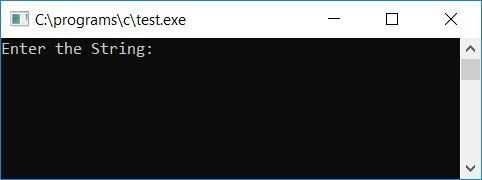Now enter any string, say "codescracker," and press the ENTER key. Then enter any character that presents itself inside the string "codescracker", say "c", and press the ENTER again to see the frequency of "c" in "codescracker."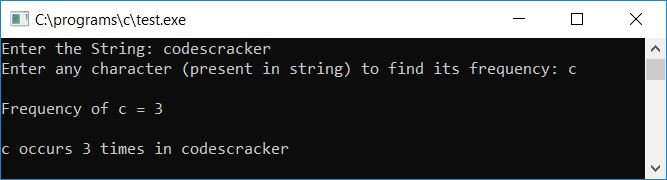#### Program Explained

1. Get string input from the user using the gets() function.
2. Get character input from the user using the scanf() function.
3. We've now created a for loop to check each and every character of the string, from the 0th to the null terminated character ('\0') of the string.
4. Whenever the character matches with the character at any place in the string, then increment the count variable's value.
5. Because the count variable was initially set to 0, it now holds the total number of times the character appears within the string.
6. For example, let's suppose the string is "codescracker" (in the str variable) and the character is "c" (in the ch variable).
7. As a result, str contains c, str contains o, str contains d, str contains e,... str contains k, str contains e, and str contains r.
8. The character c is presented at index numbers 0, 5, and 8. total of three times.
9. Therefore, the count variable gets incremented three times. Because the condition ch==str[i] is met three times, because ch holds c and str, str, and str also hold c.
10. Therefore, the frequency of c in the given string, say "codescracker," will be 3.

## Find the frequency of each character in a string

Now let's modify the above program and, instead of finding the frequency of one particular character, let's find the frequency of every character present in the given string:

```#include<stdio.h>
#include<conio.h>
#include<string.h>
int main()
{
char str, ch;
int i, j, k, len, count=0;
printf("Enter the string: ");
gets(str);
len = strlen(str);
printf("\n");
for(i=0; i<len; i++)
{
ch = str[i];
for(j=0; j<len; j++)
{
if(ch==str[j])
{
count++;
for(k=j; k<(len-1); k++)
{
str[k] = str[k+1];
}
len--;
str[len] = '\0';
j--;
}
}
printf("%c = %d times\n", ch, count);
count=0;
i--;
}
getch();
return 0;
}```

Here is the sample run: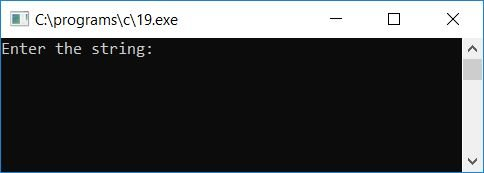Now supply any string, say "we love codescracker," and press ENTER to see the frequency of all the characters one by one as shown in the screenshot given below: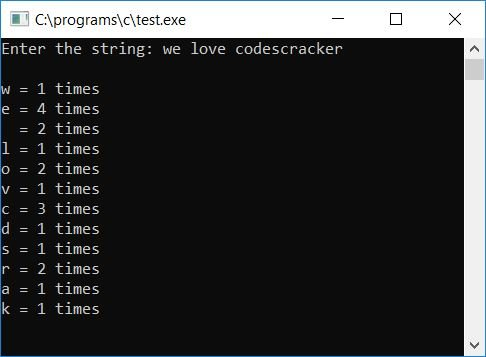#### Program Explained

1. We've used one outer loop to run until the string contains a null-terminated character ('\0').
2. And in the inner loop, we have used the same loop with another variable.
3. Before the inner loop, we've initialized the initial character of the string to the ch variable.
4. And then comes the inner loop.
5. In this loop, we checked to see if the value of ch is present anywhere in the string; if it is, we incremented the count variable and then shifted one index back all the characters of the string, so str is shifted to str, str is shifted to str, and so on.
6. As the character is counted up, therefore, the character at that particular place has no further use, so we have shifted and deleted that character from the string using the second inner for loop using another variable.
7. After exiting the loop, decrement the string length, say len, and append a null-terminated character ('\0') to the string's last index.
8. And then decrement the second inner for loop variable and continue to run until the condition of the first inner for loop evaluates to be false.
9. After this, print the output and initialize the count to 0 and decrement the value of the outer loop.
10. In this way, the frequency of all the characters is calculated and printed one by one.

### Print the frequency of each character along with the remaining characters

Here is another program that lists out all the characters' frequencies along with the remaining characters in the string:

```#include<stdio.h>
#include<conio.h>
#include<string.h>
int main()
{
char str, ch;
int i, j, k, len, count=0;
printf("Enter the string: ");
gets(str);
len = strlen(str);
printf("\n");
printf("Character\tCount\t\tString Left\n\n");
for(i=0; i<len; i++)
{
ch = str[i];
for(j=0; j<len; j++)
{
if(ch==str[j])
{
count++;
for(k=j; k<(len-1); k++)
str[k] = str[k+1];
len--;
str[len] = '\0';
j--;
}
}
printf("%c occurs \t%d times\t\t%s\n", ch, count, str);
count=0;
i--;
}
getch();
return 0;
}```

Here is its sample run:Now supply any string, say "codescracker," and press enter to check it out: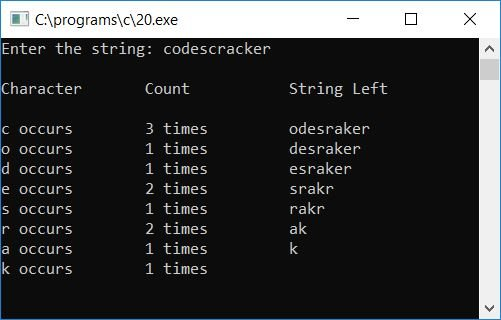#### The same program in different languages

C Quiz

« Previous Program Next Program »